# Introduction to forestploter

#### 2022-11-20

Forest plots are commonly used in the medical research publications, especially in meta-analysis. And it can also be used to report the coefficients and confidence intervals (CIs) of the regression models.

There are lots of packages out there can be used to draw a forest plot. The most popular one is forestplot. Packages specialised for the meta-analysis, like meta, metafor and rmeta. Some other packages, like ggforestplot uses ggplot2 to draw a forest plot, it is not available on the CRAN yet.

The main differences of the forestploter from the other packages are:

• Focus on the forest plot.
• Treat the forest plot as a table, elements are aligned in rows and columns. User has full control on what and how to display in the forest plot contents.
• Graphical parameters are controlled with a theme.
• Allowing post-hoc plot editing.
• CIs in multiple columns and by groups.

# Basic forest plot

The layout of the forest plot is determined by the dataset provided.

## Simple forest plot

The first step is to prepare a data.frame to be used as a basic layout of the forest plot. Column names of the data will be drawn as the header, and contents inside the data will be displayed in the forest plot. One or multiple blank columns without any content (blanks) should be provided to draw confidence interval. Width of the box to draw the CI is determined by the width of this column. Increase the number of space in the column to give more space to draw CI.

First we need to get the data ready to plot.

library(grid)
library(forestploter)

# Read provided sample example data
dt <- read.csv(system.file("extdata", "example_data.csv", package = "forestploter"))

# Keep needed columns
dt <- dt[,1:6]

# indent the subgroup if there is a number in the placebo column
dt$Subgroup <- ifelse(is.na(dt$Placebo),
dt$Subgroup, paste0(" ", dt$Subgroup))

# NA to blank or NA will be transformed to carachter.
dt$Treatment <- ifelse(is.na(dt$Treatment), "", dt$Treatment) dt$Placebo <- ifelse(is.na(dt$Placebo), "", dt$Placebo)
dt$se <- (log(dt$hi) - log(dt$est))/1.96 # Add blank column for the forest plot to display CI. # Adjust the column width with space. dt$  <- paste(rep(" ", 20), collapse = " ")

# Create confidence interval column to display
dt$HR (95% CI) <- ifelse(is.na(dt$se), "",
sprintf("%.2f (%.2f to %.2f)",
dt$est, dt$low, dt$hi)) head(dt) #> Subgroup Treatment Placebo est low hi se #> 1 All Patients 781 780 1.869694 0.13245636 3.606932 0.3352463 #> 2 Sex NA NA NA NA #> 3 Male 535 548 1.449472 0.06834426 2.830600 0.3414741 #> 4 Female 246 232 2.275120 0.50768005 4.042560 0.2932884 #> 5 Age NA NA NA NA #> 6 <65 yr 297 333 1.509242 0.67029394 2.348190 0.2255292 #> HR (95% CI) #> 1 1.87 (0.13 to 3.61) #> 2 #> 3 1.45 (0.07 to 2.83) #> 4 2.28 (0.51 to 4.04) #> 5 #> 6 1.51 (0.67 to 2.35) The data we have above will be used as basic layout of the forest plot. The example below shows how to draw a simple forest plot. A footnote was added as a demonstration. p <- forest(dt[,c(1:3, 8:9)], est = dt$est,
lower = dt$low, upper = dt$hi,
sizes = dt$se, ci_column = 4, ref_line = 1, arrow_lab = c("Placebo Better", "Treatment Better"), xlim = c(0, 4), ticks_at = c(0.5, 1, 2, 3), footnote = "This is the demo data. Please feel free to change\nanything you want.") # Print plot plot(p)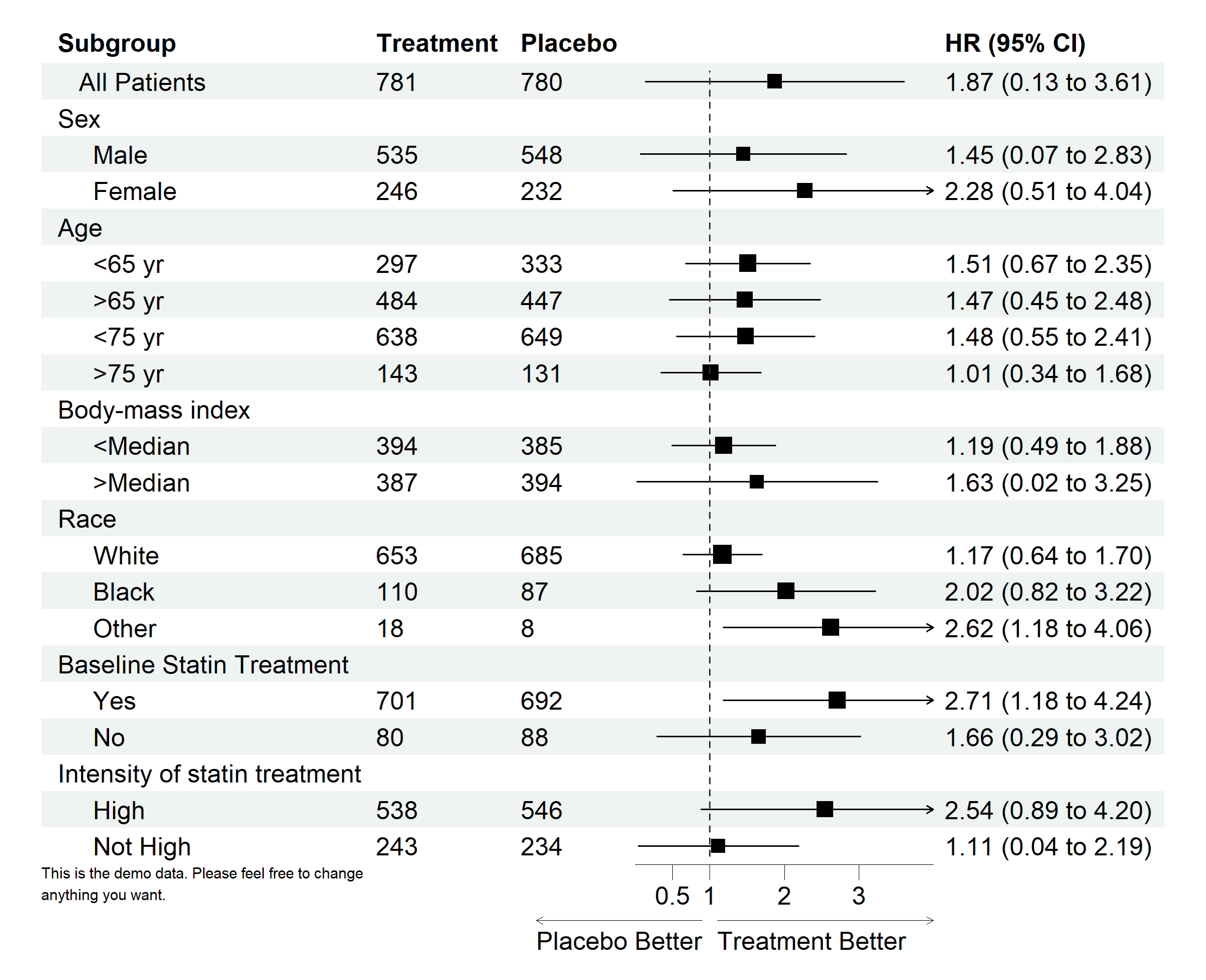## Change theme Now we will use the same data above, and add a summary point. We also want to change the graphical parameters for confidence interval and other parts of the plot. Theme of the forest plot can be adjusted with forest_theme function, check out the manual for more details. dt_tmp <- rbind(dt[-1, ], dt[1, ]) dt_tmp[nrow(dt_tmp), 1] <- "Overall" dt_tmp <- dt_tmp[1:11, ] # Define theme tm <- forest_theme(base_size = 10, # Confidence interval point shape, line type/color/width ci_pch = 15, ci_col = "#762a83", ci_fill = "black", ci_alpha = 0.8, ci_lty = 1, ci_lwd = 1.5, ci_Theight = 0.2, # Set an T end at the end of CI # Reference line width/type/color refline_lwd = 1, refline_lty = "dashed", refline_col = "grey20", # Vertical line width/type/color vertline_lwd = 1, vertline_lty = "dashed", vertline_col = "grey20", # Change summary color for filling and borders summary_fill = "#4575b4", summary_col = "#4575b4", # Footnote font size/face/color footnote_cex = 0.6, footnote_fontface = "italic", footnote_col = "blue") pt <- forest(dt_tmp[,c(1:3, 8:9)], est = dt_tmp$est,
lower = dt_tmp$low, upper = dt_tmp$hi,
sizes = dt_tmpse, is_summary = c(rep(FALSE, nrow(dt_tmp)-1), TRUE), ci_column = 4, ref_line = 1, arrow_lab = c("Placebo Better", "Treatment Better"), xlim = c(0, 4), ticks_at = c(0.5, 1, 2, 3), footnote = "This is the demo data. Please feel free to change\nanything you want.", theme = tm) # Print plot plot(pt)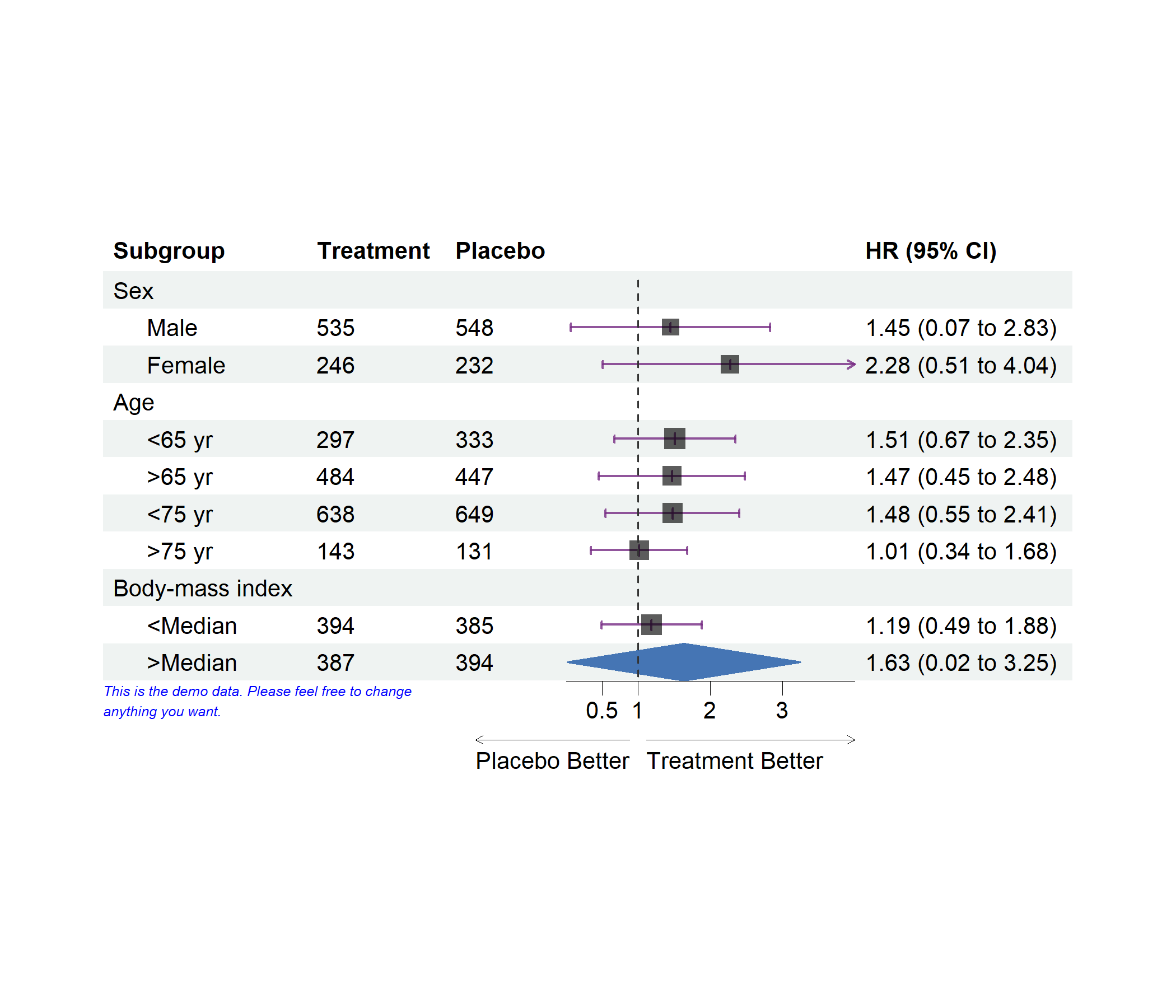## Editing forest plot The package has some functionality to modify the forestplot. Below is the functions to edit various aspects of the plot: • The edit_plot function can be used to change the graphical parameter of text, background and CI. For example the color or font face of some columns or rows. • The add_border function can be used to add a border to a specific cell. • The add_text function can be used to add text to certain rows/columns. If you want a complicated text align, you can leave some rows and/or columns blank and use this function to add the text. • The insert_text function can be used to insert a row before or after a certain row and add text. # Change text color in row 3 g <- edit_plot(p, row = 3, gp = gpar(col = "red", fontface = "italic")) # Change color of the CI g <- edit_plot(g, row = c(3, 6, 11, 13), col = 4, which = "ci", gp = gpar(col = "green")) # Bold grouping text g <- edit_plot(g, row = c(2, 5, 10, 13, 17, 20), gp = gpar(fontface = "bold")) # Edit background of row 5 g <- edit_plot(g, row = 5, which = "background", gp = gpar(fill = "darkolivegreen1")) # Insert text at top g <- insert_text(g, text = "Treatment group", col = 2:3, part = "header", gp = gpar(fontface = "bold")) # Add underline at the bottom of the header g <- add_border(g, part = "header", row = 1, where = "top") g <- add_border(g, part = "header", row = 2, where = "bottom") g <- add_border(g, part = "header", row = 1, col = 2:3, gp = gpar(lwd = 2)) # Insert text g <- insert_text(g, text = "This is a long text. Age and gender summarised above.\nBMI is next", row = 10, just = "left", gp = gpar(cex = 0.6, col = "green", fontface = "italic")) # Add border g <- add_border(g, row = 10, col = 1:3, where = "top") plot(g)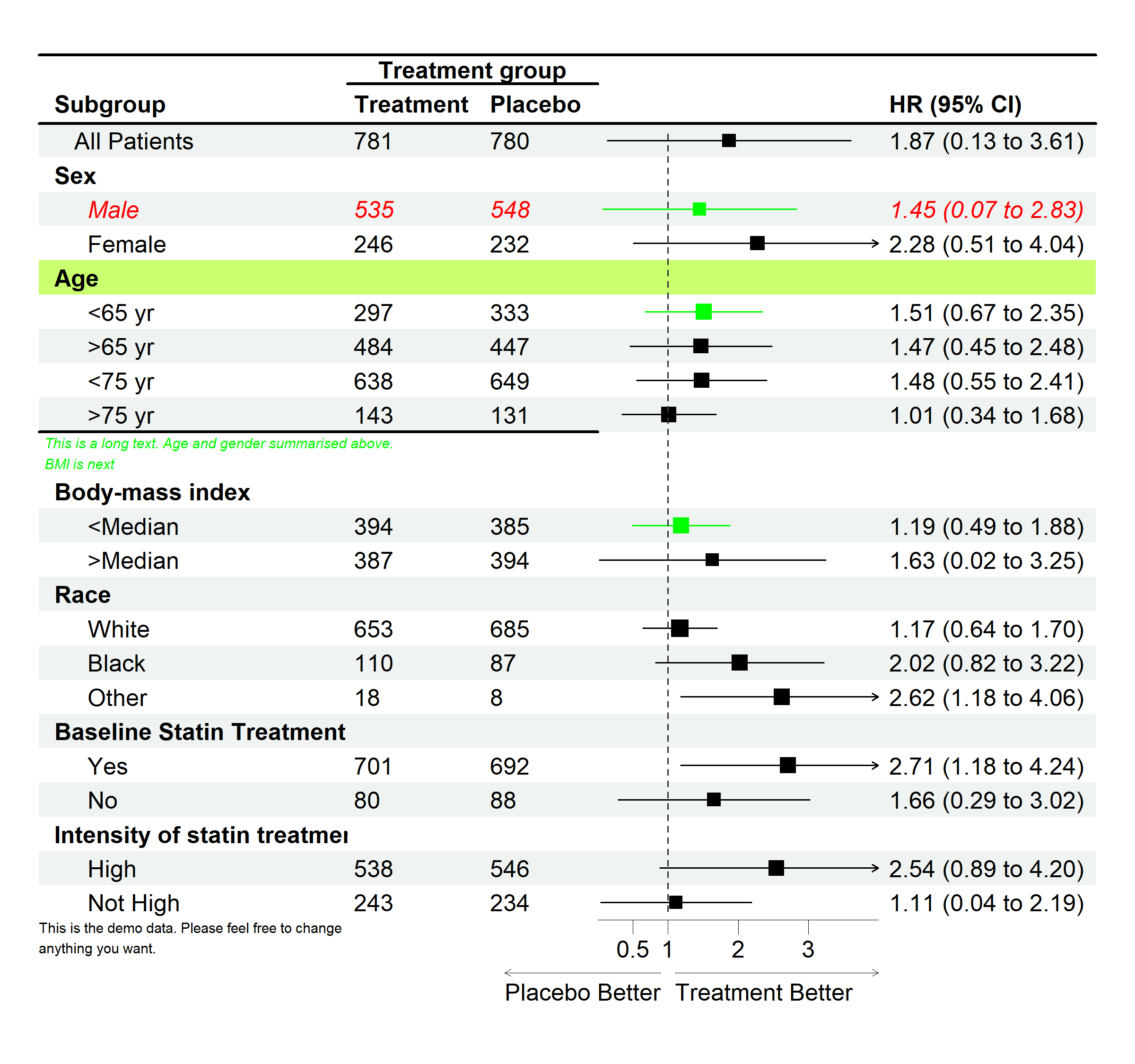The add_text function simply put the text in the plot without adding any rows to the plot. The insert_text function will insert a row and add text to the inserted row. This is the same as adding a blank row to the data before drawing a forest plot and use add_text to the row have. ## Text justification and background By default, all cells are left aligned. But it is possible to justify any cells in the forest plot by setting parameters in forest_theme. Set core=list(fg_params=list(hjust=0, x=0)) to left align content, and rowhead=list(fg_params=list(hjust=0.5, x=0.5) to center header. Set hjust=1 and x=0.9 to right align text. Same rule apply to change the background color by setting core=list(bg_params=list(fill = c("#edf8e9", "#c7e9c0", "#a1d99b"))). Change settings in core if you want to change graphical parameters of contents, colhead for header. Change settings in fg_params to modify the text, see parameters for textGrob() in grid package. Change bg_params to modify settings for background graphical parameters, see gpar() in grid package. You should pass parameters as a list. More details can be found here. Provide a single value if you want cells have the same justification or vector for each cells. As you can notice that the second example justify text by row using the provided vector, and the vector will be recycled. dt <- dt[1:4, ] # Header center and content right tm <- forest_theme(core=list(fg_params=list(hjust = 1, x = 0.9), bg_params=list(fill = c("#edf8e9", "#c7e9c0", "#a1d99b"))), colhead=list(fg_params=list(hjust=0.5, x=0.5))) p <- forest(dt[,c(1:3, 8:9)], est = dtest,
lower = dt$low, upper = dt$hi,
sizes = dt$se, ci_column = 4, title = "Header center content right", theme = tm) # Print plot plot(p)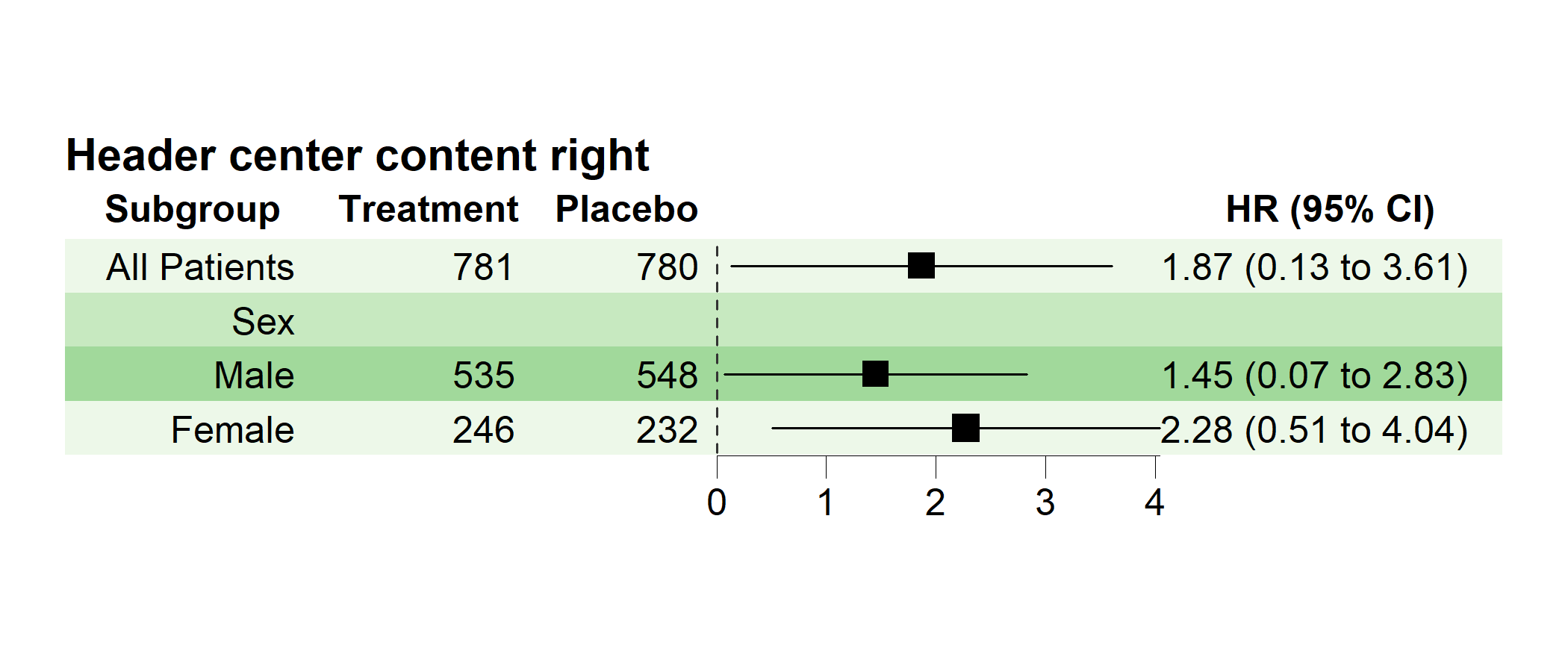# Mixed justification tm <- forest_theme(core=list(fg_params=list(hjust=c(1, 0, 0, 0.5), x=c(0.9, 0.1, 0, 0.5)), bg_params=list(fill = c("#f6eff7", "#d0d1e6", "#a6bddb", "#67a9cf"))), colhead=list(fg_params=list(hjust=c(1, 0, 0, 0, 0.5), x=c(0.9, 0.1, 0, 0, 0.5)))) p <- forest(dt[,c(1:3, 8:9)], est = dt$est,
lower = dt$low, upper = dt$hi,
sizes = dt$se, ci_column = 4, title = "Mixed justification", theme = tm) plot(p)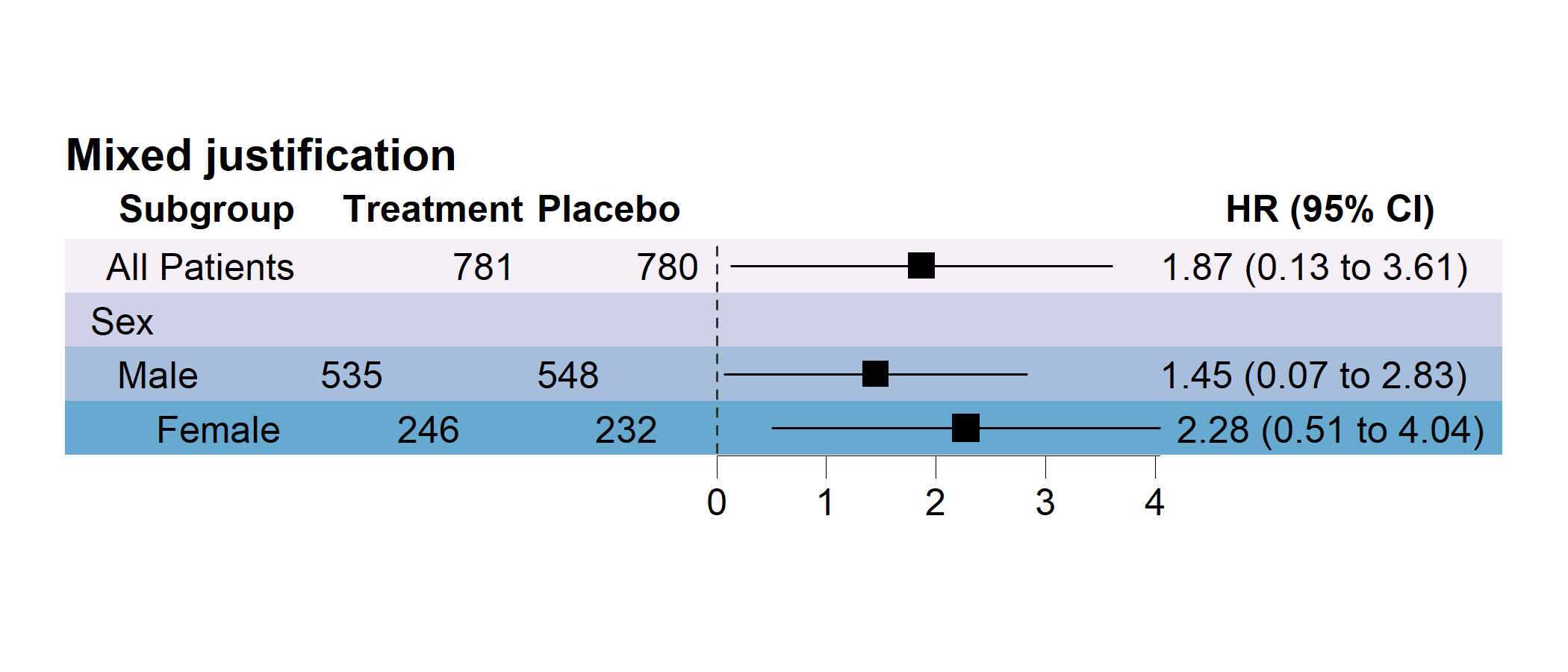# Multiple CI columns Sometimes one may want to have multiple CI columns, each column may represent different outcome. If this is the case, one only needs to provide a vector of the position of the columns to be drawn in the data. If the number of columns provided to draw the CI columns are same as number of est, one CI will be drawn into each CI columns. If the number of columns provided is less than number of est, the extra est will be considered as a group and will be drawn to the CI columns sequentially. In the latter case, group number equals to number of est devided by number of ci_column and multiple columns will be drawn into one cell. As seen in the example below, the CI will be drawn in the column 3 and 5. The first and second elements in est, lower and upper will be drawn in column 3 and column 5. In a multiple groups example, two or more CI in one cell. The solution is simple, provide all the values sequentially to est, lower and upper. Which means, the first n elements in the est, lower and upper are considered as same group, same for next n elements. The n is determined by the number of ci_column. As it is shown in the example below, est_gp1 and est_gp2 will be drawn in column 3 and column 5, considered as group 1. The est_gp3 and est_gp4 will be drawn in column 3 and column 5, considered as group 2. This is an example of multiple CI columns and groups: dt <- read.csv(system.file("extdata", "example_data.csv", package = "forestploter")) # indent the subgroup if there is a number in the placebo column dt$Subgroup <- ifelse(is.na(dt$Placebo), dt$Subgroup,
paste0("   ", dt$Subgroup)) # NA to blank or NA will be transformed to carachter. dt$n1 <- ifelse(is.na(dt$Treatment), "", dt$Treatment)
dt$n2 <- ifelse(is.na(dt$Placebo), "", dt$Placebo) # Add two blank column for CI dt$CVD outcome <- paste(rep(" ", 20), collapse = " ")
dt$COPD outcome <- paste(rep(" ", 20), collapse = " ") # Set-up theme tm <- forest_theme(base_size = 10, refline_lty = "solid", ci_pch = c(15, 18), ci_col = c("#377eb8", "#4daf4a"), footnote_col = "blue", legend_name = "Group", legend_value = c("Trt 1", "Trt 2"), vertline_lty = c("dashed", "dotted"), vertline_col = c("#d6604d", "#bababa")) p <- forest(dt[,c(1, 19, 21, 20, 22)], est = list(dt$est_gp1,
dt$est_gp2, dt$est_gp3,
dt$est_gp4), lower = list(dt$low_gp1,
dt$low_gp2, dt$low_gp3,
dt$low_gp4), upper = list(dt$hi_gp1,
dt$hi_gp2, dt$hi_gp3,
dt$hi_gp4), ci_column = c(3, 5), ref_line = 1, vert_line = c(0.5, 2), nudge_y = 0.2, theme = tm) plot(p)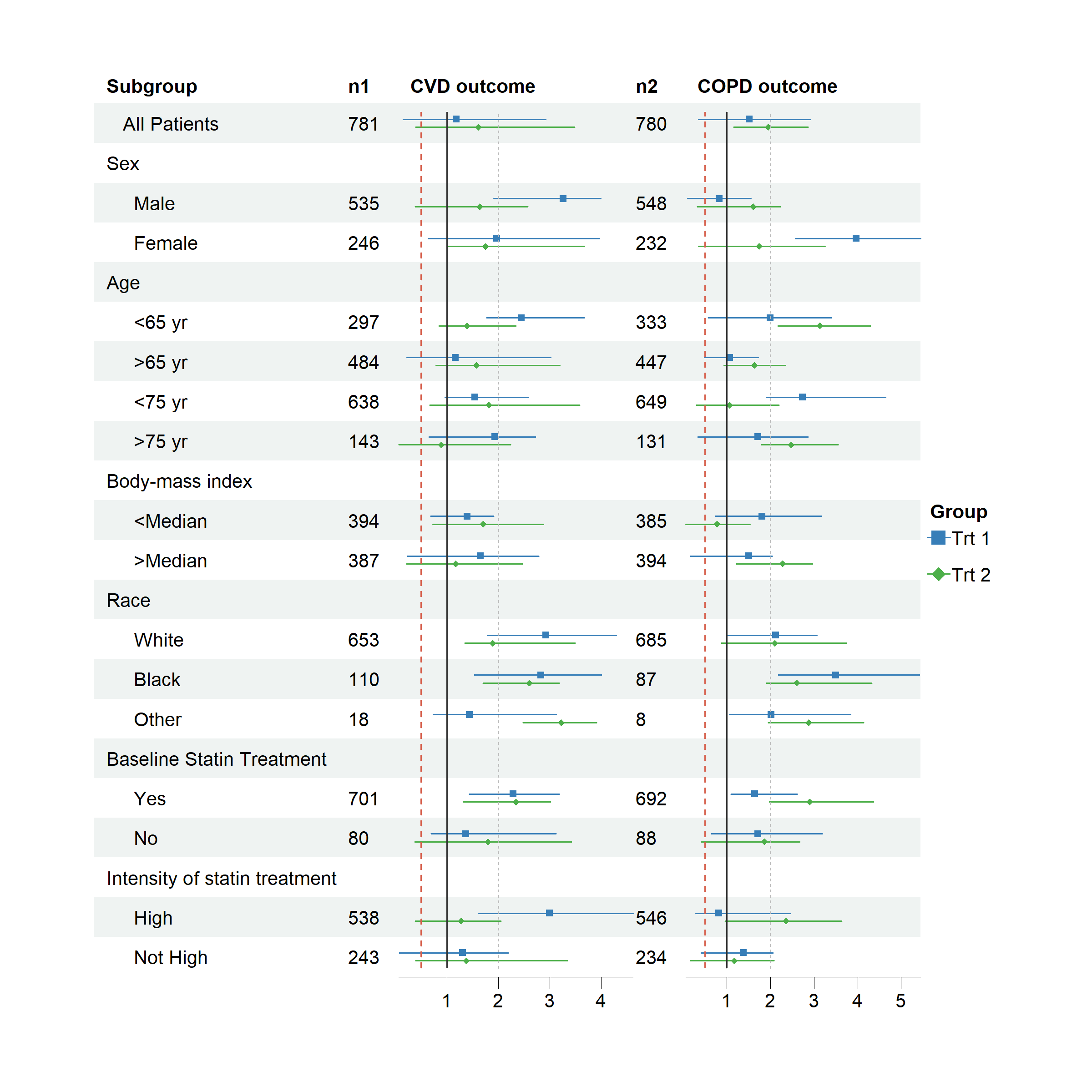# Different parameters for different CI columns If the desired forest plot is multiple column, some may want to have different settings for different columns. For example, different CI column has different xlim, x-axis ticks, x-axis labels, x_trans, reference line, vertical line or arrow labels. This can be easily done by providing a list or vector. Provide a list for xlim, vert_line, arrow_lab and ticks_at, atomic vector for xlab, x_trans and ref_line. See the example below.  dt$HR (95% CI) <- ifelse(is.na(dt$est_gp1), "", sprintf("%.2f (%.2f to %.2f)", dt$est_gp1, dt$low_gp1, dt$hi_gp1))
dt$Beta (95% CI) <- ifelse(is.na(dt$est_gp2), "",
sprintf("%.2f (%.2f to %.2f)",
dt$est_gp2, dt$low_gp2, dt$hi_gp2)) tm <- forest_theme(arrow_type = "closed", arrow_label_just = "end") p <- forest(dt[,c(1, 21, 23, 22, 24)], est = list(dt$est_gp1,
dt$est_gp2), lower = list(dt$low_gp1,
dt$low_gp2), upper = list(dt$hi_gp1,
dt$hi_gp2), ci_column = c(2, 4), ref_line = c(1, 0), vert_line = list(c(0.3, 1.4), c(0.6, 2)), x_trans = c("log", "none"), arrow_lab = list(c("L1", "R1"), c("L2", "R2")), xlim = list(c(0, 3), c(-1, 3)), ticks_at = list(c(0.1, 0.5, 1, 2.5), c(-1, 0, 2)), xlab = c("OR", "Beta"), nudge_y = 0.2, theme = tm) plot(p)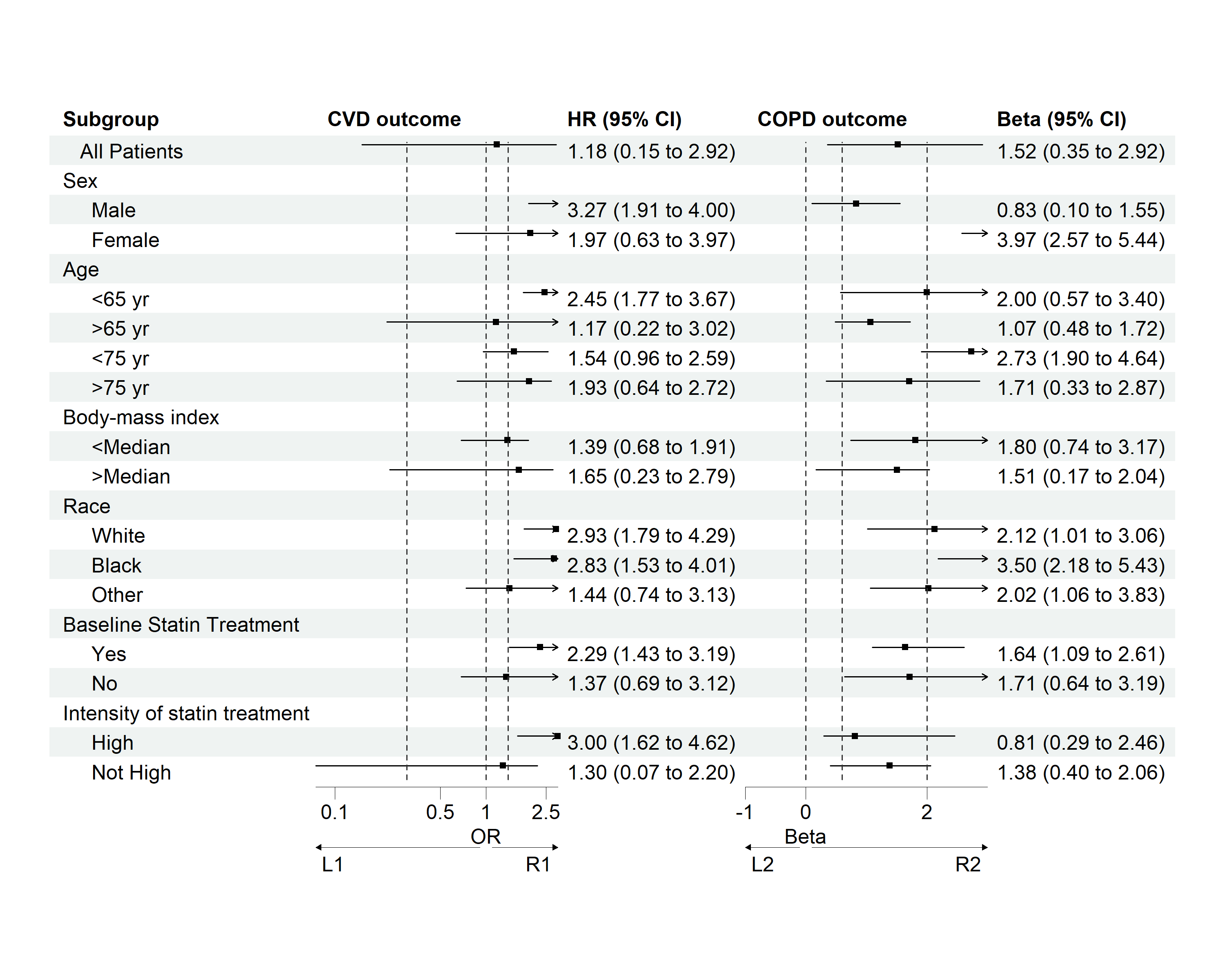# Saving plot One can use the base method or use ggsave function to save plot. For the ggsave function, please don’t ignore the plot parameter. The width and height should be tuned to get a desired plot. You can also set autofit=TRUE in the print or plot function to auto fit the plot, but this may change not be compact as it should be. # Base method png('rplot.png', res = 300, width = 7.5, height = 7.5, units = "in") p dev.off() # ggsave function ggplot2::ggsave(filename = "rplot.png", plot = p, dpi = 300, width = 7.5, height = 7.5, units = "in") Or you can get the width and height of the forestplot with get_wh, and use this width and height for saving. # Get width and height p_wh <- get_wh(plot = p, unit = "in") png('rplot.png', res = 300, width = p_wh, height = p_wh, units = "in") p dev.off() # Or get scale get_scale <- function(plot, width_wanted, height_wanted, unit = "in"){ h <- convertHeight(sum(plot$heights), unit, TRUE)
w <- convertWidth(sum(plot\$widths), unit, TRUE)
max(c(w/width_wanted,  h/height_wanted))
}
p_sc <- get_scale(plot = p, width_wanted = 6, height_wanted = 4, unit = "in")
ggplot2::ggsave(filename = "rplot.png",
plot = p,
dpi = 300,
width = 6,
height = 4,
units = "in",
scale = p_sc)